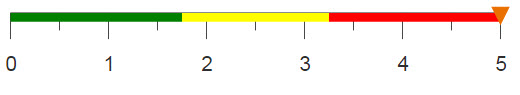# Adding source information to the description of columns

By using <loop>, you can add the path of the originating table to the description of each linked column, when combining the columns of multiple tables.

## Difficulty## Objective

You want to combine the columns of multiple tables, but you want to be able to distinguish which table each linked column originated from. You can do this by adding the path of the originating table to the description of each linked column.

## Solution

```<block ltable="pub.doc.retail.altseg.products" lcol="sku,dept,group"
lcol2="sku,dept,group">
<base table="pub.doc.retail.altseg.sales_detail_transid"/>
<set linklist="{str_to_lst(@lcol2;',')}"/>
<link table2="{@ltable}" col="{@lcol}" col2="{@lcol2}"/>
<loop with_="columns">
<outer>
<base table="{@ltable}"/>
<columns/>
<colord cols="name"/>
<transpose/>
</outer>
<inner>
<loop with_="description">
<outer>
<base table="{@ltable}"/>
<columns/>
<colord cols="desc"/>
<transpose/>
</outer>
<inner>
<letseq desc="{@description}" keys="{pkg_names(@desc)}">
<letseq names="{@columns}" keys="{pkg_names(@names)}">
<foreach text="{@keys}" colname="{@keys}" tally_="@i">
<if test="{@columns.{@colname}=@linklist.1|
@columns.{@colname}=@linklist.2|
@columns.{@colname}=@linklist.3}">
<then>
<col name="{@columns.{@colname}}"
desc="{@description.{@text}}
(column linked on)"/>
</then>
<else>
<col name="{@columns.{@colname}}"
desc="{@description.{@text}}
(originating table: {@ltable})"/>
</else>
</if>
</foreach>
</letseq>
</letseq>
</inner>
</loop>
</inner>
</loop>
</block>```

## Alternate solution

```<dynamic base="">
<do onchange_="@base" value1_="@table_names" row1_="1" col1_="1"
value2_="@column_names" row2_="1" col2_="2" value3_="@desc_text"
row3_="1" col3_="3">
<loop with_="tables">
<outer>
<directory folder="{@base}"/>
<colord cols="path"/>
<transpose/>
</outer>
<inner>
<base table="{@tables.m0}"/>
<columns/>
<willbe name="colnames" value="g_splice(;;;name;',';)"/>
<willbe name="descriptions" value="g_splice(;;;desc;',';)"/>
<willbe name="tablenames" value="'{@tables.m0}'"/>
<colord cols="tablenames,colnames,descriptions"/>
<sel value="i_=1"/>
<letseq names="{@tables}" keys="{pkg_names(@names)}">
<foreach tablename="{@keys}" tally_="@i">
<if test="{@i>1}">
<merge table2="{@tables.{@tablename}}" match="pad">
<columns/>
<willbe name="colnames" value="g_splice(;;;name;',';)"/>
<willbe name="descriptions" value="g_splice(;;;desc;',';)"/>
<willbe name="tablenames" value="'{@tables.{@tablename}}'"/>
<colord cols="tablenames,colnames,descriptions"/>
<sel value="i_=1"/>
</merge>
</if>
</foreach>
</letseq>
</inner>
</loop>
<willbe name="tables" value="g_splice(;;;tablenames;'|';)"/>
<willbe name="columns" value="g_splice(;;;colnames;'|';)"/>
<willbe name="descs" value="g_splice(;;;descriptions;'|';)"/>
<colord cols="tables,columns,descs"/>
</do>
<do onchange_="@base">
<set tablebase="{str_to_lst(@table_names;'|')}"/>
<set columnlists="{str_to_lst(@column_names;'|')}"/>
<set desclists="{str_to_lst(@desc_text;'|')}"/>
</do>
<widget class_="browser" value_="@base" filter_="folder" accept_="folder"
typevalue_="@base_type" label_="Base Path" dropwidth_="550"/>
<widget class_="text" text_="{@tablebase}"/>
<widget class_="text" text_="{@columnlists}"/>
<widget class_="text" text_="{@desclists}"/>
</dynamic>```

## Discussion

When combining columns of multiple tables, one way to be able to identify the originating table of each column is by adding a suffix to the column name. However, you can also change column metadata, such as the column description or label, using the <col> operation. This first solution in this recipe adds the path of the originating table to the description of each linked column using <col>.

In the first solution, two nested loops are used to create two packages, one for column names and one for the description of each column. Using <letseq> and <foreach> in the innermost loop, the path of the originating table is added to the description of each column. However, in the <if> statement, if the current column name in the package matches one of the linking columns (specified by col2), the phrase "(column linked on)" is amended to the description instead.

The second solution uses a browser widget in a QuickApp (within a <dynamic>) to allow the end user to select a directory folder and then generates lists of column names and descriptions for each table in the selected folder. Again, a loop is used to create a package, which contains a path for each table in the folder. In order to generate the strings of column names and descriptions, g_splice(G;S;O;X;D;N) is used on the name and desc columns in each table. The resulting columns are joined together using g_splice(G;S;O;X;D;N) to create a master string.

The first <do onchange_="@base"> construct allows you to assign the value of a particular cell (denoted by a given row and column) to a dynamic variable. Finally the strings are turned into lists using str_to_lst(X;Y).

Text widgets display the lists to ensure everything was properly executed. These lists can then be used to change the column metadata in any of the tables you decide to link from the selected folder.

## Common errors

Non-list component in compound @variable name "lcol2.1"
In the first solution, if you simply try to use @lcol2.1 in your <if> statement to denote the first column name designated to link on, you will receive this error.

Even though the column names you entered in the variable lcol2 are separated by commas, the variable as a whole is considered as a string by the system and not a list. In order to access each item in the list using an index, you must first use the str_to_lst(X;Y) function to transform the string into a list-value. Setting this to a new variable, you can now use an index to access each item (i.e., @linklist.1).

## Further reading

If you would like to learn more about the functions and operations discussed in this recipe, click on the links below:

<loop>

<link>

pkg_names(X)